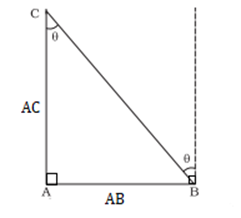Request a call back

1. EXPLAIN HOW TO MEASURE DISTANCES USING THE PARALLAX METHOD (BCZ IT IS CONFUSING). 2. HOW DID THE MOLECULAR DISTANCE HAS BEEN FOUND USING THAT ACID(WHOLE PROCEDURE)?Expert Answer

Ans 1. The parallax method is a way to measure distances by using the apparent shift of an object against a background as the observer moves. To measure the distance using the parallax method, the observer first finds the object and marks its position. The observer then moves to a different location and marks the object's new position. The distance between the two positions is then measured and used to calculate the distance to the object.

To put it simply, imagine you are standing at position A and you want to calculate the distance between you and an object at point C, as shown in the figure below.Now, in the given case, we move to point B, which is perpendicular to point A and at distance AB from its initial position, thus we can find the distance AB and angle θ.

Using these we can calculate AC by using formula.This is how we measure distance using parallax method.

Note: Please elaborate the second question in detail, so that we can provide the solution for the same.

Answered by Jayesh Sah | 17 Oct, 2022, 08:21: AM
CBSE 11-science - Physics
if the momentum of body decreases by 30% its kinetic energy decreased byAsked by bhargavchary19 | 23 Dec, 2022, 07:33: AMANSWERED BY EXPERT
CBSE 11-science - PhysicsANSWERED BY EXPERT
CBSE 11-science - Physics
Asked by kdimple765 | 09 Jun, 2022, 05:07: PMANSWERED BY EXPERT
CBSE 11-science - Physics
Asked by rajveermundel7 | 18 May, 2022, 09:01: PMANSWERED BY EXPERT
CBSE 11-science - Physics
Asked by duritanaik | 03 May, 2022, 08:59: AMANSWERED BY EXPERT
CBSE 11-science - Physics
Asked by imayushanand223 | 29 Dec, 2021, 08:49: AMANSWERED BY EXPERT
CBSE 11-science - Physics
Asked by vithalsawant119 | 24 Nov, 2021, 09:06: PMANSWERED BY EXPERT
CBSE 11-science - Physics
Asked by anupchan2005 | 03 Sep, 2021, 10:20: PMANSWERED BY EXPERT
CBSE 11-science - Physics
Asked by bamanetushar48 | 29 May, 2021, 02:16: PMANSWERED BY EXPERT
CBSE 11-science - Physics
Asked by aartiingole27 | 31 Mar, 2021, 02:26: PMANSWERED BY EXPERT